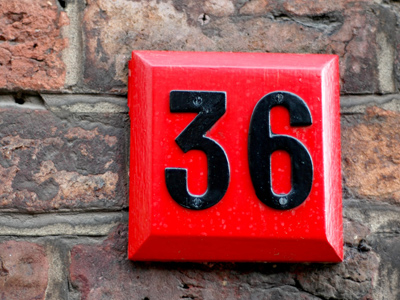6 × 6 = 36.

# 6 Times Table

Learning the six times table - a must for the 11-Plus - involves multiplying numbers by 6. There are various ways of doing this. Taking 8 x 6 as an example, we could multiply the 8 by 5 to get 40 and then add another 8 to reach 48. But that takes a little time. Learning the times tables off by heart will things a lot easier for you!

Six is a number you'll come across a lot. Insects such as flies, ants, beetles and wasps have six feet. There are six feet in a fathom. A fathom is a nautical measure of depth: it equals approximately 1.8 m. Volleyball and ice hockey are both played with teams of 6 players. A cube, also known as a hexahedron, has six faces, that's why there are six numbers on most dice.

Be careful when you pick your answers. If you rush you might hit the wrong one - and you wouldn't want that now would you? Practise makes perfect so keep coming back to this quiz even when you've mastered it.

1.
6 × 2 = ?
12
10
16
9
To multiply a number by 2 just add it to itself
2.
6 × 8 = ?
28
38
48
58
One way to multiply a number by 8 is to double it, then double your answer and finally double it again
3.
6 × 3 = ?
16
18
24
15
To work this out you could first multiply 3 x 5 and then add 3
4.
6 × 4 = ?
34
14
44
24
6 x 5 = 30 and 30 - 6 = 24
5.
6 × 5 = ?
40
20
30
35
To multiply a number by 5, first times it by 10 and then half your answer
6.
6 × 7 = ?
32
22
52
42
In the comic sci-fi novel The Hitchhiker's Guide to the Galaxy, the answer to the ultimate question of life, the universe and everything is 42
7.
6 × 6 = ?
36
46
26
56
36 is 6 squared, so 6 is the square root of 36
8.
6 × 11 = ?
66
65
64
63
To multiply a number by 11, first times it by 10 and then add the first number
9.
6 × 12 = ?
65
72
75
82
The exterior angles of a pentagon measure 72 degrees
10.
6 × 9 = ?
54
34
64
74
6 x 10 = 60 and 60 - 6 = 54
Author:  Frank Evans